# How to Generate Random Numbers in Excel (Ultimate Guide)

Random numbers are those numbers which are generated by a process in which the outcome is not predictable and in a defined interval/set, the values are uniformly distributed. Random numbers are used in statistical sampling, computer simulations, data encryption, lottery and in the areas where unpredictable results are desired. In this article, we will see many processes on how to generate random numbers in excel. We will also see the procedures of generating letters, words, date and time also.

## Basic use of RAND function in Excel

Rand function is used to generate random numbers. If we use it directly it generates random numbers between zero and one.

Whenever you edit your worksheet, new numbers are generated in the area where RAND functions are placed. The reason of this is, RAND functions are a volatile function.

RAND function doesn`t have any arguments. You can enter the =RAND () function in any cell and copy/drag the formula to use it in other cells.

The following picture shows the basic use of RAND function where many random numbers are generated.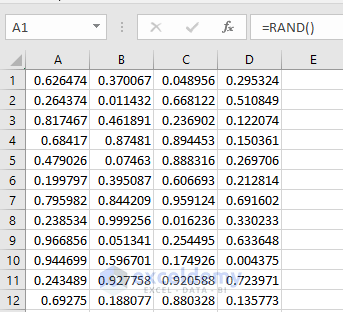## Uses of RAND function with a range

Normally RAND function generates random numbers which are between zero and one. But if you want to produce random numbers between two values you need to modify the RAND function.

### Specifying the upper limit

In this way, you can produce random numbers from 0 too N where N is the upper limit in this case. You just need to multiply the function RAND by N.

Let’s say we want to generate some random number from 0 too 25. We will multiply 25 with the RAND function. It will produce random numbers in a sequence which are greater than/equal to zero but less than 25.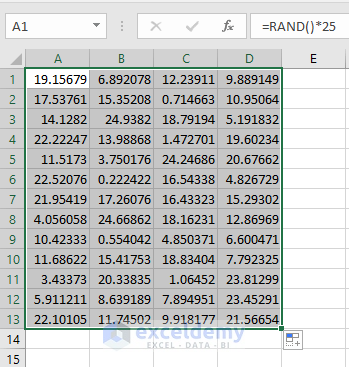Note: In excel the upper limit is never included in the sequence of random numbers. If you want to generate numbers between 0 and 4 including 4, you need to specify the upper limit as 5.

### Generating random numbers between two numbers

The previous example shows us the random numbers which are between 0 and any value N. A question might arise that “How can we create random numbers between two values that don’t start with zero? Well, we can use a formula to execute that which is,

RAND () *(Y-X) +X

Where Y is the highest value and X is the lowest value in the range.

The following picture shows an example of generating arbitrary numbers between 100 and 50.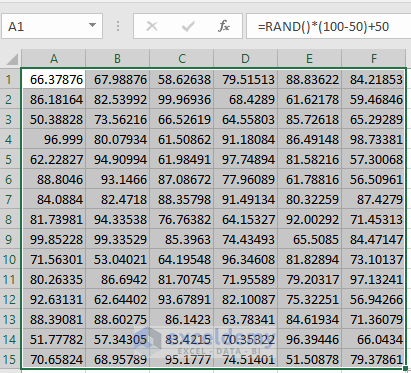Note: This formula never returns a number which is the largest is a specific range.

### Generating Excel random integer with RAND function

To create random integer numbers in excel we can use the above two formulas wrapping in INT function.

For creating random integers which are between 0 & 25, we can use the formula

=INT (RAND () *25)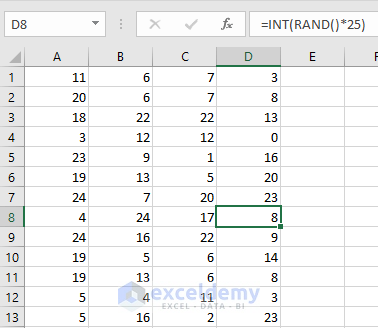For creating arbitrary integers between 100 and 50, we can use the formula

=INT (RAND () *(50-10) +10)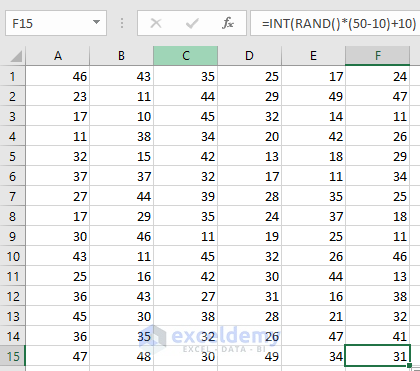## Use of RANDBETWEEN function

In the previous examples, we create random numbers in between two numbers with RAND function. But it is more useful to use RANDBETWEEN functions instead of RAND function in this case. RANDBETWEEN function returns a random number that is between a bottom and top range. Like RAND function RANDBETWEEN is also a volatile function and it will generate new random numbers whenever you edit your worksheet.

### Generating random integer with RANDBETWEEN function in Excel

The interesting thing about using the RANDBETWEEN function is that generally, it will produce integer random number whenever you are using it. Even with the decimal range, it will always generate integer random numbers. To generate numbers with a decimal in the RANDBETWEEN function, you have to edit the general formula.

For instance, if you want to create random integers in between 15 and 30 (including 10 and 50), The following formula can be used.
=RANDBETWEEN (15, 30)

The following picture shows us the result of this example.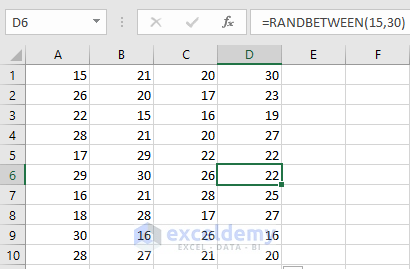### Generating random numbers with decimal places

As stated before, RANDBETWEEN function is designed to produce random integer numbers in excel, but by some little modification, it can also be used to produce random decimal numbers.

Let’s work with the previous example where we got random integer numbers from 15 too 30. Here instead of getting the integer numbers if we want to get the decimal numbers, the modification of the formula will be:

=RANDBETWEEN(15*10,30*10)/10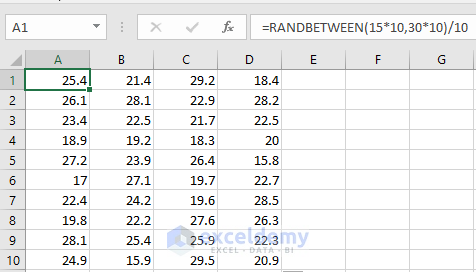The above picture shows us the numbers with 1 decimal place. If we want to get random numbers with 2 decimal places the formula will be

=RANDBETWEEN(15*100,30*100)/100

## Random letter generator in Excel

To produce random letters in excel a combination of three different functions are required. Let’s say we want to create random letters from A to Z. We will use the formula as stated below:

=CHAR(RANDBETWEEN(CODE(“A”),CODE(“Z”)))

In the above formula:

• Firstly, numeric ANSI codes for the specified letters are returned by the function CODE.
• The numbers returned by the CODE functions are taken by the RANDBETWEEN function as the upper and lower values of the range.
• Finally, corresponding letters are converted by CHAR function (CHAR converts random ANSI codes returned by RANDBETWEEN)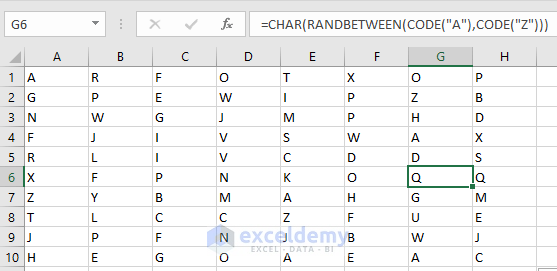Note: The above formula is case sensitive as the ANSI code is different for upper-case and lower-case characters.

## Generate random names in excel from a list

Generating random names in Excel is so much interesting. You can literally play lottery by doing it. In this example we will be using a column consists of 15 names. One name will be selected randomly from that column/ list. The formula for doing this is given below.

=INDEX(\$A\$1:\$A\$14,RANDBETWEEN(1,14))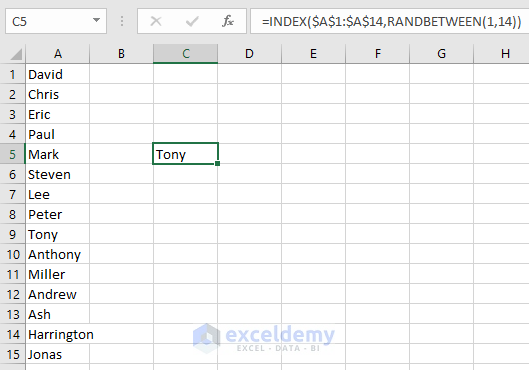Here the given range is taken as \$A\$1: \$A\$14 from the 15 names in column A. From this range we want to take a random name from the top 14 names. RANDBETWEEN (1,14) allows us to take random 14 names for the operation. The INDEX function allows returning a value from the given range. By dragging the formulated cell in upward and downward we can copy the formula for whose cells.

Note: It is mandatory to specify the random name range (which is stated in the RANDBETWEEN function here). If the specified random name range is not in the main name range (Here \$A\$1: \$A\$14) then it may sometimes ask for references.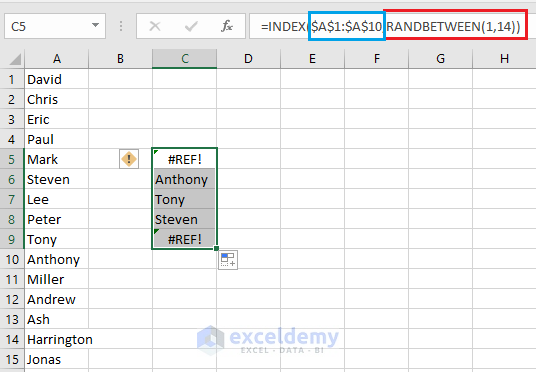As you can see. The main name range is given as \$A\$1: \$A\$10 but the specified name range (1,14) from which the names will be generated are not in this range. So, it will ask for references as stated in the above picture.

## Random dates generator in Excel

To produce some arbitrary dates between two dates, we can use the RANDBETWEEN function with DATEVALUE. Here in this example, we generate some dates between 1st March 2018 to 17th March 2018. The Formula is given below.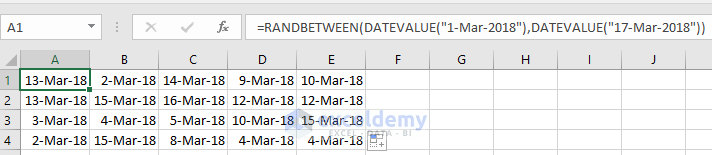Note: Sometimes the cells may not give you date rather it will give you some random number. You need to format the cell in specific date format to show dates in the cells.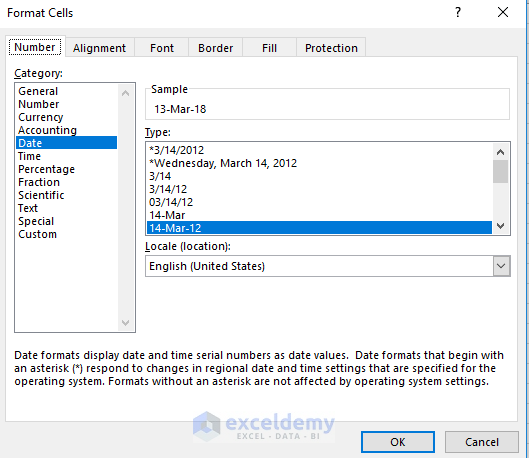## Random time generator in Excel

The procedure of generating arbitrary times between two times is same as above. The formula can be given as

=TIMEVALUE(“Starting Time”) + RAND() * (TIMEVALUE(“Ending Time”) – TIMEVALUE(“Starting Time”))

Here in this example, we use,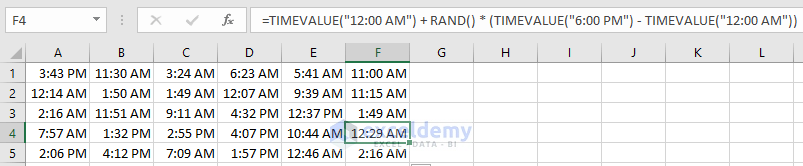Same thing can be written as,

=TIME(Starting Time) + RAND() * (TIME(Ending Time) – TIME(Starting Time))

Here in this case the formula will be,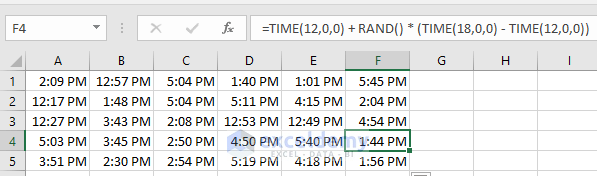Note: The TIMEVALUE function actually gives a valid time from a text string and TIME function gives time with hours, minutes, and seconds. If you again find a random number instead of time, format the cells with specific time format.

## Generating Random Dates & Times together

To do this, we need to combine RANDBETWEEN, DATEVALUE and TIMEVALUE functions together. Here we used the following formula to create dates and times together in a range.

 Source code`=RANDBETWEEN(DATEVALUE("1-Mar-2018"),DATEVALUE("17-Mar-2018"))+RANDBETWEEN(TIMEVALUE("8:00 AM")*10000,TIMEVALUE("8:00 PM")*10000)/10000`

Where the start and end dates are 1-Mar-2018 & 17-Mar-2018 respectively. The start and end times are 8:00 AM & 8:00 PM respectively.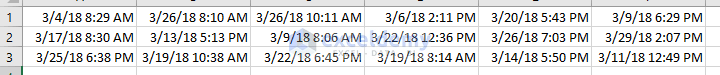## Unique random number generator in Excel (with no duplicates/repeats)

Generating random numbers are easy but when it comes to generating unique random numbers the task is complicated. There are so many methods that we can perform. In this article, we will demonstrate some of the methods available for generating unique random numbers.

### Sorting a list of unique numbers in random order

In this method, we will create a list of numbers in serial order. Then again in another column, we will use the RAND function. The two columns, then, will be filtered and we will see that the 1st column where the numbers are in serial order will be distributed randomly. As the numbers that we provided are not repeated here, we will find a list of unique numbers in a column.

• 1st make two columns titled with Number and Random. In the number, column creates a series of 15 numbers in serial order starting from 1. To do this click on the Fill option above and in that click on A box will come up, select columns in there. Write 1 as Step value and 15 as Stop value. Then press OK.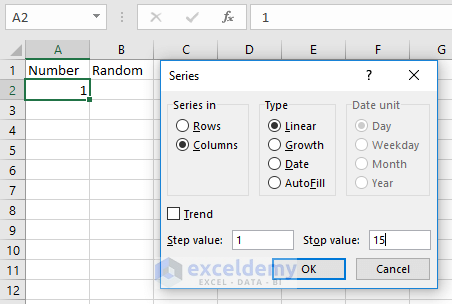• In the Random column create a list of arbitrary numbers. Make sure to create the column in the same range with the 1st column
• After that click on the Sort & Filter option and click on to Filter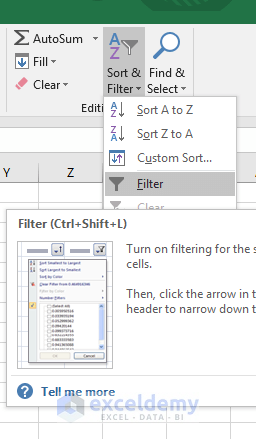• In the Random column click any two option Sort Smallest to Largest/Largest to smallest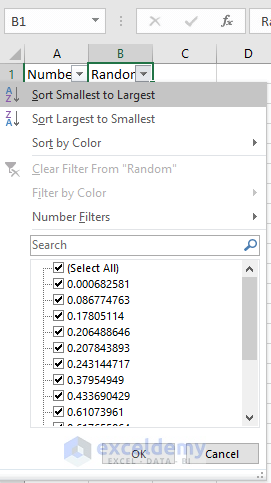• You will see, by doing the sorting the numbers in the 1st columns will change their places randomly from one cell to another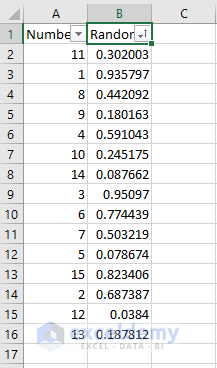### Ranking random integers

• 1st create some random numbers in a list, let’s say create 20 random numbers in column A.
• IN cell B1 type the formula =RANK(A1,A\$1:A\$20) and drag this formula to B20 cell. You will see that in column B 20 unique random numbers will be generated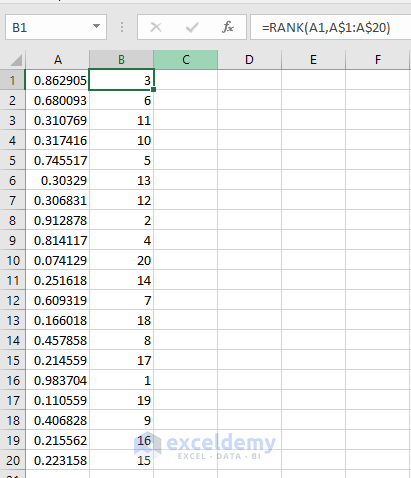### Using the largest values and the MATCH function

• 1st create some random numbers in a list, let’s say create 10 random numbers in column A.
• In cell B1, write the formula =MATCH(LARGE(\$A\$1:\$A\$20, ROW(A1)), \$A\$1:\$A\$10, 0) and drag the formula from B1 to B10. It will unique random numbers from 1 to 10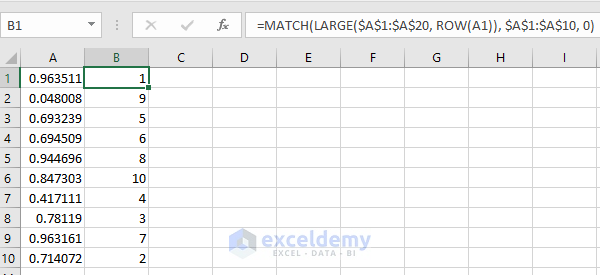Note: You can use COLUMN instead of ROW. In that case, you have to drag the formulated cell in right/left side. If you use ROW in the formula and drag it to left/right it will produce the same numbers.

### Using the RAND and INDEX function

• 1st create a list of arbitrary numbers, let’s say create 10 random numbers in column A.
• In column B, create 10 Random integer numbers and located them through B1 to B10
• Use the formula =INDEX(A\$1: A\$10, RANK(B1, B\$1: B\$10)) in cell C1 and drag it down to cell C10. You will see 10 unique random numbers will appear in column D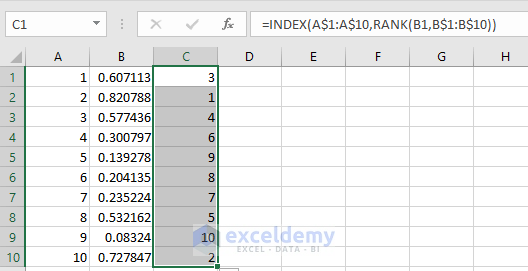## Excel VBA random number generator with no duplicates

Generating unique random number is more efficient in VBA code than using different functions of excel. In excel, there are so many VBA codes available to generate numbers which can be unique or repeated. In this example, I generate 10 arbitrary numbers using VBA code.

The code that I used here was collected from http://access-excel.tips/generate-non-duplicated-random-number/ this link.

I found it very useful, short and effective among all the codes that I found while doing the task.

The code is given below.

`Public Sub generateRandNum()`

`    lowerbound = 1`

`    upperbound = 10`

`    Set randomrange = Range("A1:A10")`

`    randomrange.Clear`

`    For Each rng1 In randomrange`

`        counter = counter + 1`

`    Next`

`    If counter > upperbound - lowerbound + 1 Then`

`        MsgBox ("Number of cells > number of unique random numbers")`

`        Exit Sub`

`    End If`

`    For Each Rng In randomrange`

`        randnum = Int((upperbound - lowerbound + 1) * Rnd + lowerbound)`

`        Do While Application.WorksheetFunction.CountIf(randomrange, randnum) >= 1`

`            randnum = Int((upperbound - lowerbound + 1) * Rnd + lowerbound)`

`        Loop`

`        Rng.Value = randnum`

`    Next`

`End Sub`

To use this code, follow the following steps.

• Click on the Developer tab
• From Code group, select Visual Basic
• Enter the above code in the worksheet module and run it.

You will see that 10 numbers from 1-10 appear in column A. You can modify it by just changing the upper bound, lower bound and range. The below picture shows the result of the above code.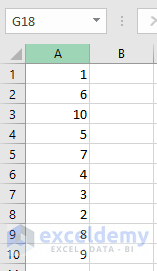## Conclusion

Random numbers are useful in so many ways. As we can see there are so many methods of generating random numbers. With the existing RAND and RANDBETWEEN functions, you can not generate unique random numbers directly. Some modifications are required while generating unique random numbers.

#### Siam Hasan Khan

Hello! Welcome to my Profile. Here I will be posting articles related to Microsoft Excel. I have completed my BSc in Electrical and Electronic Engineering from American International University-Bangladesh. I am a diligent, goal-oriented engineer with an immense thirst for knowledge and attitude to grow continuously. Continuous improvement and life-long learning is my motto.

Mallory Stevens March 21, 2018 at 9:16 PM

Thanks for another great blog post! I can’t wait to share it with my Excel students. I always learn something new from your post.

I found a small typo in Generating Excel random integer with RAND function. You have 50, instead of 25, as the multiplier (correct in the photo).

Thanks again for sharing!

Siam Hasan March 27, 2018 at 6:34 PM

Thanks for identifying the typing mistake. I have solved it already. It`s good to know that you find this article useful.

Cheers!!!